# Team:Shanghai Metro Utd/Model

Shanghai_Metro_Utd

MODEL
As known from the litterateurs that Heparan sulfate Proteoglycans (HSPGs) are needed for rANG to enter cells, it is necessary to explore the specific combination of ANG and HS interaction. Therefore, we applied an enzyme-linked immunosorbent assay to detect the bonding strength between rANG and HS and further analyzed the relationship by building a mathematical model.
Below is the initial data. The OD450 could indicate the interaction ability between heparin and angiogenin which means that the higher the OD450 value, the greater the interaction ability between heparin and angiogenin.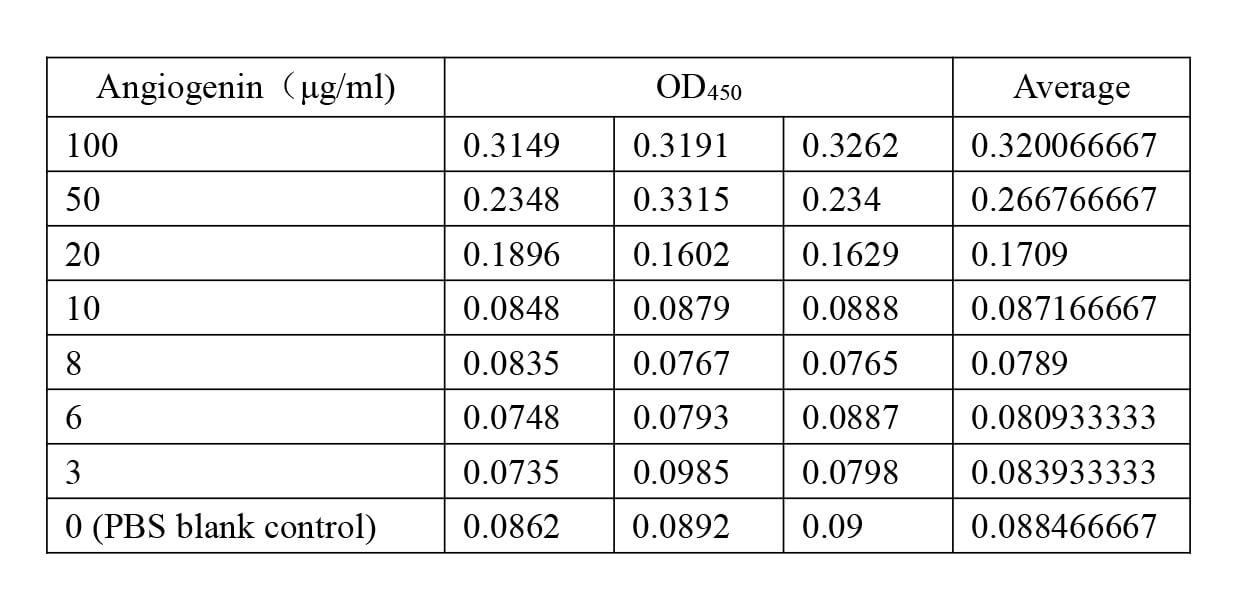According to the scatter plots, there seemed several ups and downs during 3~8 ug/ml which could be resulted from the lower concentration and the instrument errors, but the basic trend of the data presents an S trend. In this case, we chose the logistic equation to fit our data: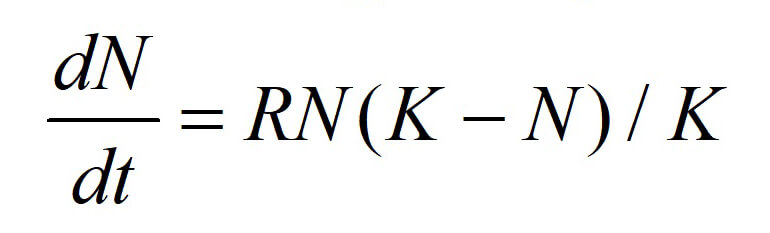After importing the data in MATLAB, we received the modeling results below where the fitting degree is 0.9833.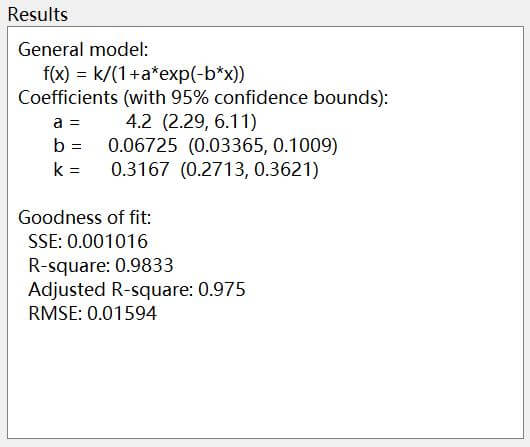Figure 1. Model result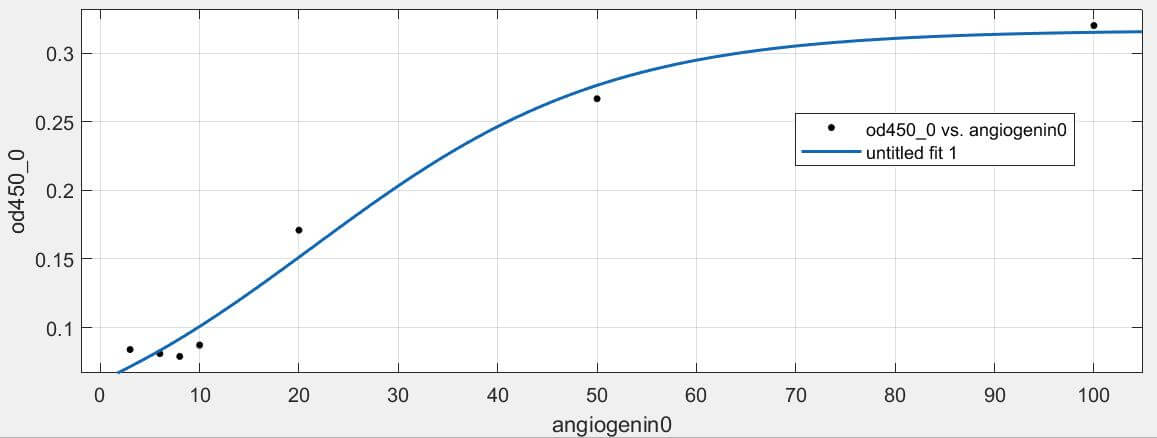Figure 2. The fitting curve of the model
Based on the model result and fitting curve, it indicates that the more angiogenin we added, the higher the OD450 value, namely the interaction ability between heparin and angiogenin. Therefore, it can be concluded that the interaction of heparin has dose-dependent with angiogenin and conforms to a certain quantitative relation (Fig. 1). This model could contribute to the development of new drugs related to angiogenin.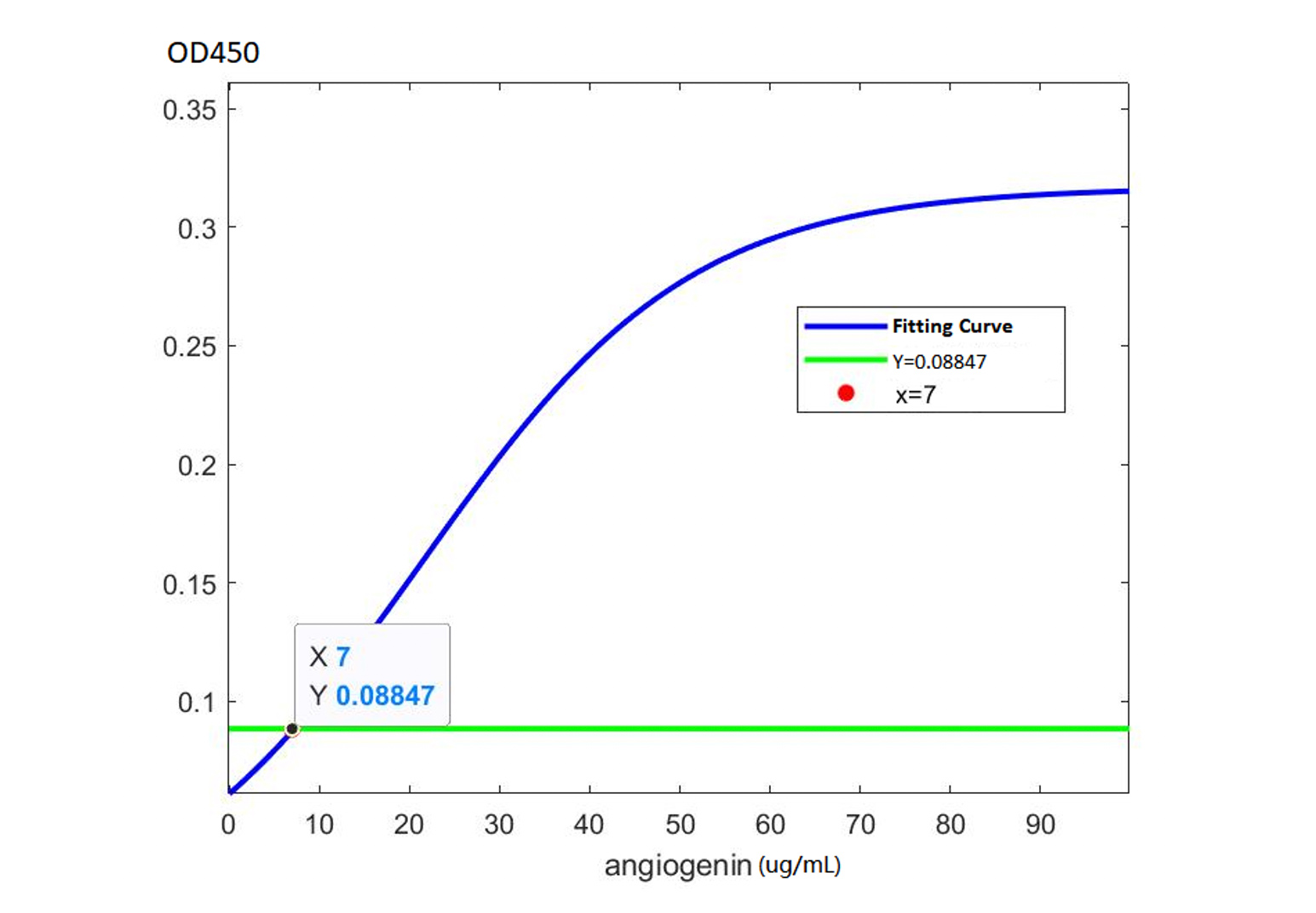Figure 3. Comparison graph to the PBS blank control
In order to determine the least amount of angiogenin that could combine with heparin, we introduced a blank control, PBS solution as the comparison where its OD450 value’s average equals to 0.08847. In this case, we drew a horizontal line (Y=0.008847) into the fitting curve graph of the model (Fig. 3) and we could find the cross point at (7, 0.08847). Therefore, it could be inferred that the least amount of angiogenin that could combine with heparin would be 7 ug/ml.